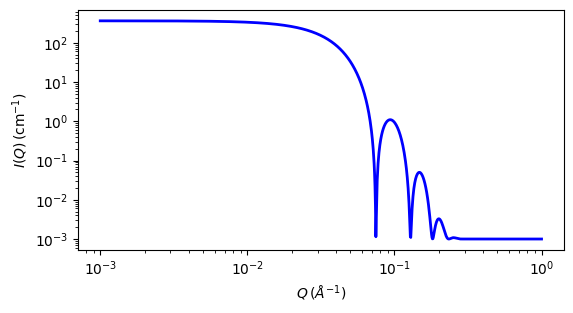# fuzzy_sphere

Scattering from spherical particles with a fuzzy surface.

Parameter

Description

Units

Default value

scale

Scale factor or Volume fraction

None

1

background

Source background

cm-1

0.001

sld

Particle scattering length density

10-6-2

1

sld_solvent

Solvent scattering length density

10-6-2

3

60

fuzziness

std deviation of Gaussian convolution for interface (must be << radius)

10

The returned value is scaled to units of cm-1 sr-1, absolute scale.

For information about polarised and magnetic scattering, see the Polarisation/Magnetic Scattering documentation.

Definition

The scattering intensity $$I(q)$$ is calculated as:

$I(q) = \frac{\text{scale}}{V}(\Delta \rho)^2 A^2(q) S(q) + \text{background}$

where the amplitude $$A(q)$$ is given as the typical sphere scattering convoluted with a Gaussian to get a gradual drop-off in the scattering length density:

$A(q) = \frac{3\left[\sin(qR) - qR \cos(qR)\right]}{(qR)^3} \exp\left(\frac{-(\sigma_\text{fuzzy}q)^2}{2}\right)$

Here $$A(q)^2$$ is the form factor, $$P(q)$$. The scale is equivalent to the volume fraction of spheres, each of volume, $$V$$. Contrast $$(\Delta \rho)$$ is the difference of scattering length densities of the sphere and the surrounding solvent.

Poly-dispersion in radius and in fuzziness is provided for, though the fuzziness must be kept much smaller than the sphere radius for meaningful results.

From the reference:

The “fuzziness” of the interface is defined by the parameter $$\sigma_\text{fuzzy}$$. The particle radius $$R$$ represents the radius of the particle where the scattering length density profile decreased to 1/2 of the core density. $$\sigma_\text{fuzzy}$$ is the width of the smeared particle surface; i.e., the standard deviation from the average height of the fuzzy interface. The inner regions of the microgel that display a higher density are described by the radial box profile extending to a radius of approximately $$R_\text{box} \sim R - 2 \sigma$$. The profile approaches zero as $$R_\text{sans} \sim R + 2\sigma$$.

For 2D data: The 2D scattering intensity is calculated in the same way as 1D, where the $$q$$ vector is defined as

$q = \sqrt{{q_x}^2 + {q_y}^2}$Fig. 80 1D plot corresponding to the default parameters of the model.

Source

fuzzy_sphere.py $$\ \star\$$ fuzzy_sphere.c $$\ \star\$$ sas_3j1x_x.c

References

1. M Stieger, J. S Pedersen, P Lindner, W Richtering, Langmuir, 20 (2004) 7283-7292

Authorship and Verification

• Author: